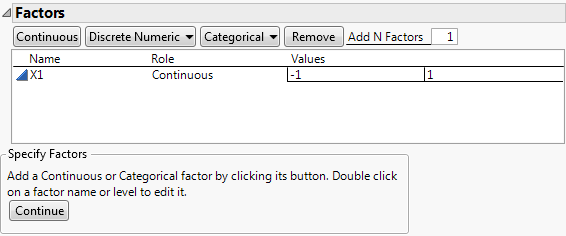Publication date: 11/10/2021

## Factors

Add factors in the Factors outline.

Tip: When you have completed the Factors outline, consider selecting Save Factors from the red triangle menu. This option saves the factor names, roles, changes, and values in a data table that you can later reload in DOE platforms.

Figure 9.14 Factors OutlineContinuous

Adds a Continuous factor. The data type in the resulting data table is Numeric. A continuous factor is a factor that you can conceptually set to any value between the lower and upper limits you supply, given the limitations of your process and measurement system.

Discrete Numeric

Adds a Discrete Numeric factor. A discrete numeric factor can assume only a discrete number of numeric values. These values have an implied order. The data type in the resulting data table is Numeric.

A screening design includes all levels of a discrete numeric factor and attempts to balance the levels. Fit Model treats a discrete numeric factor as a continuous predictor.

The default values for a discrete numeric factor with k levels, where k > 2, are the integers 1, 2, ..., k. The default values for a discrete numeric factor with k = 2 levels are -1 and 1. Replace the default values with the settings that you plan to use in your experiment.

Categorical

Adds a Categorical factor. Click to select or specify the number of levels. The data type in the resulting data table is Character. The value ordering of the levels is the order of the values, as entered from left to right. This ordering is saved in the Value Order column property after the design data table is created.

The default values for a categorical factor are L1, L2,..., Lk, where k is the number of levels that you specify. Replace the default values with level names that are relevant for your experiment.

Remove

Removes the selected factors.

Adds multiple factors. Enter the number of factors to add, click Add Factor, and then select the factor type. Repeat Add N Factors to add multiple factors of different types.

### Factors Outline

The Factors outline contains the following columns:

Name

The name of the factor. When added, a factor is given a default name of X1, X2, and so on. To change this name, double-click it and enter the desired name.

Role

The Design Role of the factor. The Design Role column property for the factor is saved to the data table. This property ensures that the factor type is modeled appropriately. The Role of the factor determines other factor properties that are saved to the data table. See Factor Column Properties.

Values

The experimental settings for the factors.

#### Editing the Factors Outline

In the Factors outline, note the following:

To edit a factor name, double-click the factor name.

To edit a value, click the value in the Values column.

### Factor Column Properties

For each factor, various column properties are saved to the design table after you create the design by selecting Make Table in the Screening Design window. These properties are also saved automatically to the data table that is created when you select the Save Factors option. You can find more information about these column properties and related examples in Column Properties.

Coding

If the Role is Continuous or Discrete Numeric, the Coding column property for the factor is saved. This property transforms the factor values so that the low and high values correspond to –1 and +1, respectively. See Coding.

Value Order

If the Role is Categorical or if a Block variable is constructed, the Value Order column property for the factor is saved. This property determines the order in which levels of the factor appear. See Value Order.

Design Role

Each factor is given the Design Role column property. The Role that you specify in defining the factor determines the value of its Design Role column property. When you select a design with a block, that Block factor is assigned the Blocking value. The Design Role property reflects how the factor is intended to be used in modeling the experimental data. Design Role values are used in the Augment Design platform. See Design Role.

Factor Changes

Each factor is assigned the Factor Changes column property with the value of Easy. The Factor Changes property reflects how the factor is used in modeling the experimental data. Factor Changes values are used in the Augment Design and Evaluate Design platforms. See Factor Changes.

RunsPerBlock

For a blocking factor, indicates the maximum allowable number of runs in each block. When a Blocking factor is specified in the Factors outline, the RunsPerBlock column property is saved for that factor. See RunsPerBlock.